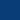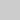## Parallelizing tree edit distance

### (master thesis or bachelor project)

The tree edit distance (TED) is a commonly used measure for comparing rooted ordered labelled trees. TED is defined as the minimum number of simple node operations (delete, insert, relabel) that transform one tree into another. The first recursive formula for TED has been introduced in the late 1970's by Tai. Since then, several algorithms implementing that formula have been proposed. The recent and most efficient development is the RTED algorithm by Pawlik and Augsten (2011). Unfortunately, Demaine et al. have shown that all algorithms that implement the recursive formula by Tai have at least cubic runtime in the worst case.

Despite the simplicity and meaningfulness of the measure, TED is sometimes disregarded due to its inefficiency (due to cubic runtime and quadratic memory complexities, comparing trees of one million nodes may require 100 hours runtime and 1TB memory). One way of improving the efficiency of an algorithm is to distribute the computation to multiple CPU cores. However, parallelizing the tree edit distance is far from being trivial. TED is computed using dynamic programming, where the results of smaller problems are used to calculate the values for larger problems. In order to parallelize the algorithm, the dependencies between these values must be considered.

Until now, there are only two research publications proposing parallel solutions for TED. The goal of this thesis is to study these approaches and develop new techniques for TED which reduce both, runtime and memory requirements. In particular, the following tasks are foreseen:

• study the literature concerning the tree edit distance and its parallel computation,
• implement the parallel TED algorithms from literature and measure their performance compared to the non-parallel versions,
• design new techniques to solve TED on multiple CPUs with an emphasis on memory consumption.

Contact: Mateusz Pawlik, Willi Mann, Nikolaus Augsten

AlgorithmikProgrammierungBenchmarkingInnovation